• 如果有一个整型变量count，多个线程并发让count自增1，你会怎么设计？
• 你知道如何让多个线程协作完成一件事件吗？

## 前言

ConcurrentHashMap是个老生常谈的集合类了，我们都知道多线程环境下不能直接使用HashMap，而需要使用ConcurrentHashMap，但有没有了解过ConcurrentHashMap到底是如何实现线程安全的呢？他到底跟传统的Hashtable和SynchronizeMap（没听过SynchronizeMap？他就是Collections.synchronizeMap方法返回的对象）到底好在哪？

ConcurrentHashMap建立在HashMap的基础上实现了线程安全，关于HashMap读者可以参考这篇文章：深入剖析HashMap，从散列表的三大要素：哈希函数、哈希冲突、扩容方案、以及线程安全展开详解HashMap的设计。关于HashMap的内容本文不再赘述，读者若对HashMap的底层设计不了解，一定要先去阅读前面的文章。ConcurrentHashMap中蕴含的并发编程智慧是非常值得我们学习的，正如文章开头的两个问题，你会如何解决呢？可能会直接上锁或用更高性能的CAS，但ConcurrentHashMap给了我们更不一样的解决方案。

## CAS与自旋锁

CAS是ConcurrentHashMap中的一个重点，也是ConcurrentHashMap提升性能的根基所在。在阅读源码中，可以发现CAS无处不在。在介绍ConcurrentHashMap前，必须先介绍一下这两个重点。

Java中的运算并不是原子操作，如`count++`可分为：

1. 获取count副本count_
2. 对count_进行自增
3. 把count_赋值给count

CAS的思路并不复杂。还是上面的例子：当我们需要对变量count进行自增时，我们可以认为没有发生并发冲突，先存储一个count副本，再对count进行自增，然后把副本和count本身进行比较，如果两者相同，则证明没有发生并发冲突，修改count的值；如果不同，则说明count在我们自增的过程中被修改了，把上述整个过程重新来一遍，直到修改成功为止，如下图：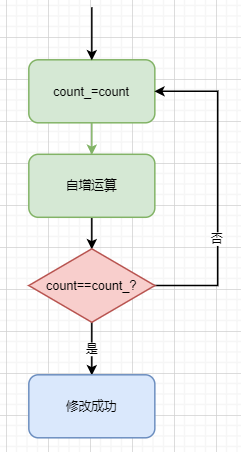``````// 比较并替换
U.compareAndSwapInt();
U.compareAndSwapLong();
U.compareAndSwapObject();``````

``````// 0代表锁释放，1代表锁被某个线程拿走了
int lock = 0;

while(true){
if(lock==0){
int lock_ ;
if(U.compareAndSwapInt(this,lock_,0,1)){
... // 获取锁后的逻辑处理

// 最后释放锁
lock = 0;
break;
}
}
}``````

CAS和自旋锁在ConcurrentHashMap应用地非常广泛，在源码中我们会经常看到他们的身影。同时这也是ConcurrentHashMap的设计核心所在。

## ConcurrentHashMap的并发策略概述

Hashtable与SynchronizeMap采取的并发策略是对整个数组对象加锁，导致性能及其低下。jdk1.7之前，ConcurrentHashMap采用的是锁分段策略来优化性能，如下图：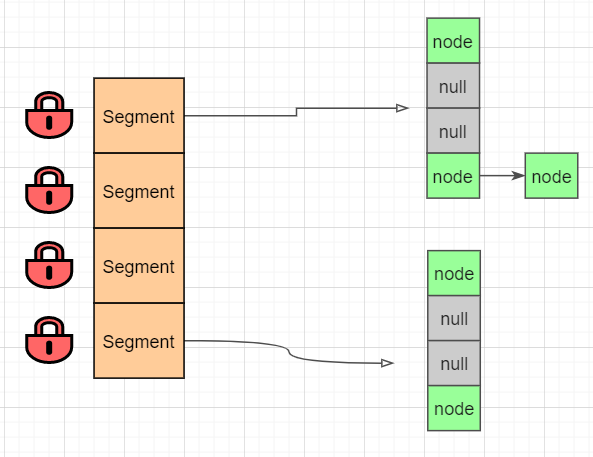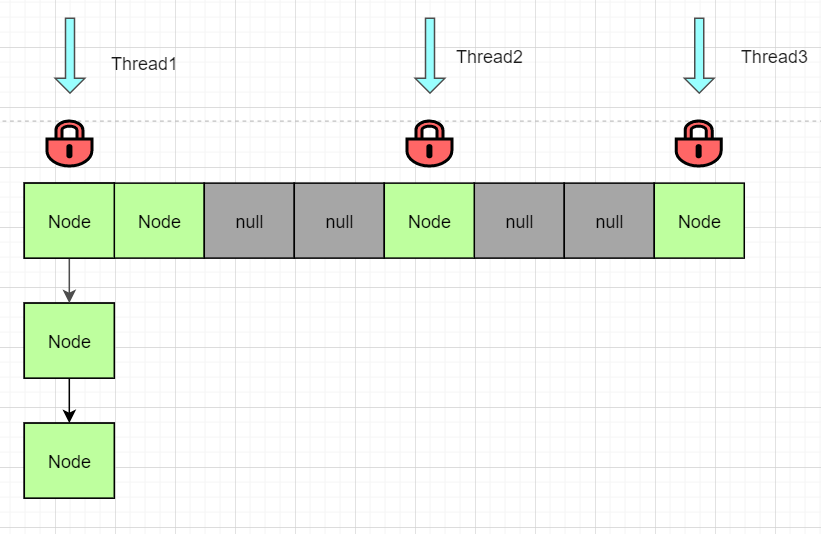## 添加数据：putVal()

ConcurrentHashMap添加数据时，采取了CAS+synchronize结合策略。首先会判断该节点是否为null，如果为null，尝试使用CAS添加节点；如果添加失败，说明发生了并发冲突，再对节点进行上锁并插入数据。在并发较低的情景下无需加锁，可以显著提高性能。同时只会CAS尝试一次，也不会造成线程长时间等待浪费cpu时间的情况。

ConcurrentHashMap的put方法整体流程如下（并不是全部流程）：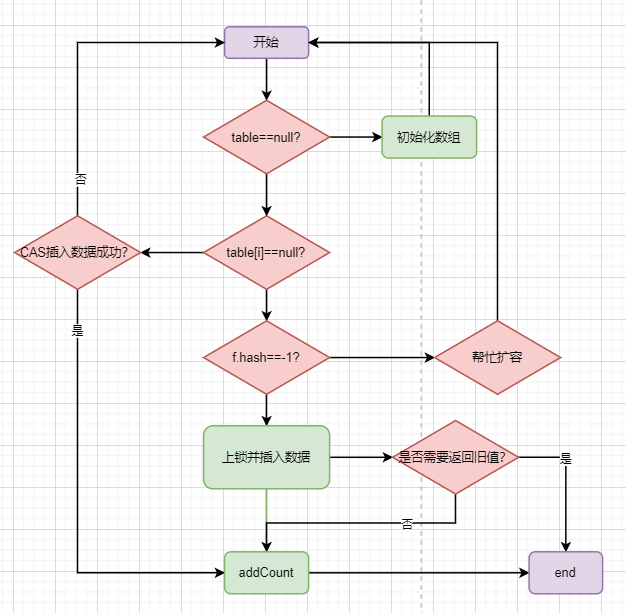1. 首先会判断数组是否已经初始化，如若未初始化，会先去初始化数组；
2. 如果当前要插入的节点为null，尝试使用CAS插入数据；
3. 如果不为null，则判断节点hash值是否为-1；-1表示数组正在扩容，会先去协助扩容，再回来继续插入数据。（协助扩容后面会讲）

ConcurrentHashMap是基于HashMap改造的，其中的插入数据、hash算法和HashMap都大同小异，这里不再赘述。思路清晰之后，下面我们看源码分析：

``````final V putVal(K key, V value, boolean onlyIfAbsent) {
// 不允许插入空值或空键
// 允许value空值会导致get方法返回null时有两种情况：
// 1. 找不到对应的key2. 找到了但是value为null；
// 当get方法返回null时无法判断是哪种情况，在并发环境下containsKey方法已不再可靠，
// 需要返回null来表示查询不到数据。允许key空值需要额外的逻辑处理，占用了数组空间，且并没有多大的实用价值。
// HashMap支持键和值为null，但基于以上原因，ConcurrentHashMap是不支持空键值。
if (key == null || value == null) throw new NullPointerException();
// 高低位异或扰动hashcode，和HashMap类似
// 但有一点点不同，后面会讲,这里可以简单认为一样的就可以
// bincount表示链表的节点数
int binCount = 0;
// 尝试多种方法循环处理，后续会有很多这种设计
for (Node<K,V>[] tab = table;;) {
Node<K,V> f; int n, i, fh;
// 情况一：如果数组为空则进行初始化
if (tab == null || (n = tab.length) == 0)
tab = initTable();
// 情况二：目标下标对象为null
else if ((f = tabAt(tab, i = (n - 1) & hash)) == null) {
// 重点：采用CAS进行插入
if (casTabAt(tab, i, null,new Node<K,V>(hash, key, value, null)))
break;
}
// 情况三：数组正在扩容，帮忙迁移数据到新的数组
// 同时会新数组，下次循环就是插入到新的数组
// 关于扩容的内容后面再讲，这里理解为正在扩容即可
else if ((fh = f.hash) == MOVED)
tab = helpTransfer(tab, f);
// 情况四：直接对节点进行加锁，插入数据
// 下面代码很多，但逻辑和HashMap插入数据大同小异
// 因为已经上锁，不涉及并发安全设计
else {
V oldVal = null;
// 同步加锁
synchronized (f) {
// 重复检查一下刚刚获取的对象有没有发生变化
if (tabAt(tab, i) == f) {
// 链表处理情况
if (fh >= 0) {
binCount = 1;
// 循环链表
for (Node<K,V> e = f;; ++binCount) {
K ek;
// 找到相同的则记录旧值
if (e.hash == hash &&
((ek = e.key) == key ||
(ek != null && key.equals(ek)))) {
oldVal = e.val;
// 判断是否需要更新数值
if (!onlyIfAbsent)
e.val = value;
break;
}
Node<K,V> pred = e;
// 若未找到则插在链表尾
if ((e = e.next) == null) {
pred.next = new Node<K,V>(hash, key,
value, null);
break;
}
}
}
// 红黑树处理情况
else if (f instanceof TreeBin) {
Node<K,V> p;
binCount = 2;
if ((p = ((TreeBin<K,V>)f).putTreeVal(hash, key,
value)) != null) {
oldVal = p.val;
if (!onlyIfAbsent)
p.val = value;
}
}
else if (f instanceof ReservationNode)
throw new IllegalStateException("Recursive update");
}
}
// 判断是否需要转化为红黑树，和返回旧数值
if (binCount != 0) {
if (binCount >= TREEIFY_THRESHOLD)
treeifyBin(tab, i);
if (oldVal != null)
return oldVal;
break;
}
}
}
// 总数+1；这是一个非常硬核的设计
// 这是ConcurrentHashMap设计中的一个重点，后面我们详细说
return null;
}

// 这个方法和HashMap
static final int spread(int h) {
return (h ^ (h >>> 16)) & HASH_BITS;
}``````

## 初始化数组：initTable()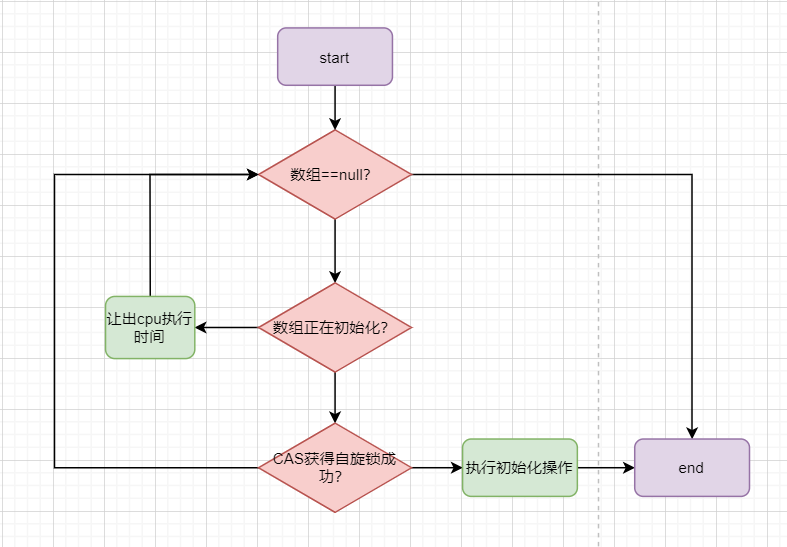1. 首先会判断数组是否为null，如果否说明另一个线程初始化结束了，直接返回该数组；
2. 第二步判断是否正在初始化，如果是会让出cpu执行时间，当前线程自旋等待
3. 如果数组为null，且没有另外的线程正在初始化，那么会尝试获取自旋锁，获取成功则进行初始化，获取失败则表示发生了并发冲突，继续循环判断。

ConcurrentHashMap并没有直接采用上锁的方式，而是采用CAS+自旋锁的方式，提高了性能。自旋锁保证了只有一个线程能真正初始化数组，同时又无需承担synchronize的高昂代价，一举两得。在看源码分析之前，我们先来了解一下ConcurrentHashMap中一个关键的变量：sizeCtl

`sizeCtl`默认为0，在正常情况下，他表示ConcurrentHashMap的阈值，是一个正数。当数组正在扩容时，他的值为-1，表示当前正在初始化，其他线程只需要判断`sizeCtl==-1` ，就知道当前数组正在初始化。但当ConcurrentHashMap正在扩容时，sizeCtl是一个表示当前有多少个线程正在协助扩容的负数 ，我们下面讲到扩容时再分析。我们直接来看`initTable()`的源码分析：

``````private final Node<K,V>[] initTable() {
Node<K,V>[] tab; int sc;
// 这里的循环是采用自旋的方式而不是上锁来初始化
// 首先会判断数组是否为null或长度为0
// 没有在构造函数中进行初始化，主要是涉及到懒加载的问题
while ((tab = table) == null || tab.length == 0) {
// sizeCtl是一个非常关键的变量；
// 默认为0，-1表示正在初始化，<-1表示有多少个线程正在帮助扩容，>0表示阈值
if ((sc = sizeCtl) < 0)

// 通过CAS设置sc为-1，表示获得自选锁
// 其他线程则无法进入初始化，进行自选等待
else if (U.compareAndSwapInt(this, SIZECTL, sc, -1)) {
try {
// 重复检查是否为空
if ((tab = table) == null || tab.length == 0) {
int n = (sc > 0) ? sc : DEFAULT_CAPACITY;
@SuppressWarnings("unchecked")
Node<K,V>[] nt = (Node<K,V>[])new Node<?,?>[n];
table = tab = nt;
// 设置sc为阈值，n>>>2表示1/4*n，也就相当于0.75n
sc = n - (n >>> 2);
}
} finally {
// 把sc赋值给sizeCtl
sizeCtl = sc;
}
break;
}
}
// 最后返回tab数组
return tab;
}``````

ConcurrentHashMap并不是一个单独的size变量，他把size进行拆分，如下图：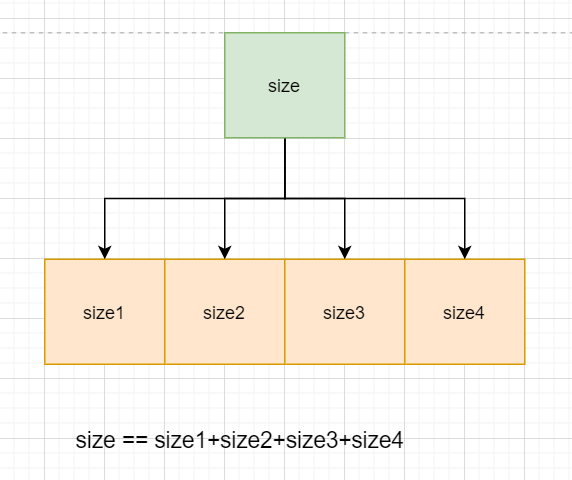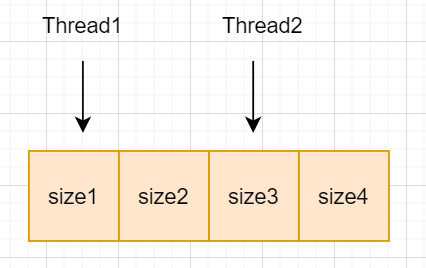``````static final class CounterCell {
volatile long value;
CounterCell(long x) { value = x; }
}``````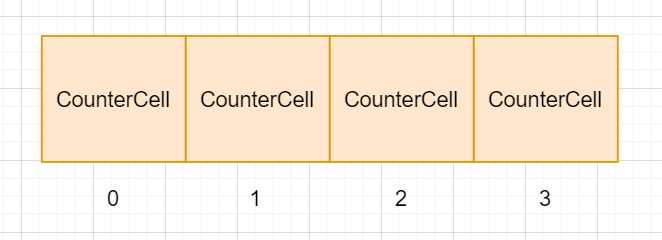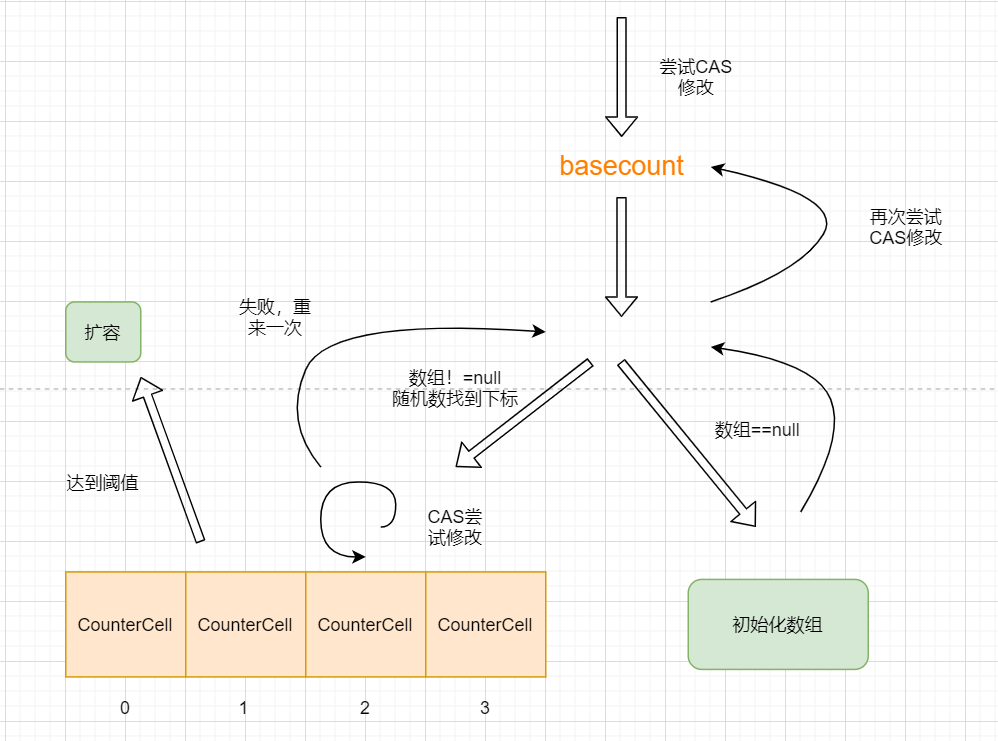1. 当前线程首先会使用CAS修改basecount的值，修改失败则进入数组分配CounterCell修改；
2. 判断CounterCell数组是否为空，
1. 如果CounterCell数组为空，则初始化数组
2. 如果CounterCell数组不为空，使用线程随机数找到下标
1. 如果该下标的的counterCell对象还没初始化，则先创建一个CounterCell，这一步在图中我没有标出来。创建了CounterCell之后还需要考虑是否需要数组扩容
2. 如果counterCell对象不为null，使用CAS尝试修改，失败则重新来一次
3. 如果上面两种情况都不满足，则会回去再尝试CAS修改一下basecount

``````private final void addCount(long x, int check) {
CounterCell[] as; long b, s;
// 如果数组不为空 或者 数组为空且直接更新basecount失败
if ((as = counterCells) != null ||
!U.compareAndSwapLong(this, BASECOUNT, b = baseCount, s = b + x)) {

CounterCell a; long v; int m;
// 表示没发生竞争
boolean uncontended = true;
// 1. 数组为null且直接修改basecount失败
// 2. hash后的数组下标CounterCell对象为null
// 3. CAS修改CounterCell对象失败
if (as == null || (m = as.length - 1) < 0 ||
(a = as[ThreadLocalRandom.getProbe() & m]) == null ||
!(uncontended =
U.compareAndSwapLong(a, CELLVALUE, v = a.value, v + x))) {
// 该方法保证完成更新，重点方法！！
return;

}

// 如果长度<=1不需要扩容（说实话我觉得这里有点奇怪）
if (check <= 1)
return;
s = sumCount();
}
if (check >= 0) {
// 扩容相关逻辑，下面再讲
}
}``````

``````private final void fullAddCount(long x, boolean wasUncontended) {
int h;
// 如果当前线程随机数为0，强制初始化一个线程随机数
// 这个随机数的作用就类似于hashcode，不过他不需要被查找
// 下面每次循环都重新获取一个随机数，不会让线程都堵在同一个地方
if ((h = ThreadLocalRandom.getProbe()) == 0) {
// wasUncontended表示没有竞争
// 如果为false表示之前CAS修改CounterCell失败，需要重新获取线程随机数
wasUncontended = true;
}

// 直译为碰撞，如果他为true，则表示需要进行扩容
boolean collide = false;

// 下面分为三种大的情况：
// 1. 数组不为null，对应的子情况为CAS更新CounterCell失败或者countCell对象为null
// 2. 数组为null，表示之前CAS更新baseCount失败，需要初始化数组
// 3. 第二步获取不到锁，再次尝试CAS更新baseCount
for (;;) {
CounterCell[] as; CounterCell a; int n; long v;

// 第一种情况：数组不为null
if ((as = counterCells) != null && (n = as.length) > 0) {
// 对应下标的CounterCell为null的情况
if ((a = as[(n - 1) & h]) == null) {
// 判断当前锁是否被占用
// cellsBusy是一个自旋锁，0表示没被占用
if (cellsBusy == 0) {
// 创建CounterCell对象
CounterCell r = new CounterCell(x);
// 尝试获取锁来添加一个新的CounterCell对象
if (cellsBusy == 0 &&
U.compareAndSwapInt(this, CELLSBUSY, 0, 1)) {
boolean created = false;
try {
CounterCell[] rs; int m, j;
// recheck一次是否为null
if ((rs = counterCells) != null &&
(m = rs.length) > 0 &&
rs[j = (m - 1) & h] == null) {
rs[j] = r;
// created=true表示创建成功
created = true;
}
} finally {
// 释放锁
cellsBusy = 0;
}
// 创建成功也就是+1成功，直接返回
if (created)
break;
// 拿到锁后发现已经有别的线程插入数据了
// 继续循环，重来一次
continue;
}
}
// 到达这里说明想创建一个对象，但是锁被占用
collide = false;
}
// 之前直接CAS改变CounterCell失败，重新获取线程随机数，再循环一次
else if (!wasUncontended)       // CAS already known to fail
wasUncontended = true;      // Continue after rehash
// 尝试对CounterCell进行CAS
else if (U.compareAndSwapLong(a, CELLVALUE, v = a.value, v + x))
break;
// 如果发生过扩容或者长度已经达到虚拟机最大可以核心数，直接认为无碰撞
// 因为已经无法再扩容了
// 所以并发线程数的理论最高值就是NCPU
else if (counterCells != as || n >= NCPU)
collide = false;            // At max size or stale
// 如果上面都是false，说明发生了冲突，需要进行扩容
else if (!collide)
collide = true;
// 获取自旋锁，并进行扩容
else if (cellsBusy == 0 &&
U.compareAndSwapInt(this, CELLSBUSY, 0, 1)) {
try {
if (counterCells == as) {// Expand table unless stale
// 扩大数组为原来的2倍
CounterCell[] rs = new CounterCell[n << 1];
for (int i = 0; i < n; ++i)
rs[i] = as[i];
counterCells = rs;
}
} finally {
// 释放锁
cellsBusy = 0;
}
collide = false;
// 继续循环
continue;
}

// 这一步是重新hash，找下一个CounterCell对象
// 上面每一步失败都会来到这里获取一个新的随机数
}

// 第二种情况：数组为null，尝试获取锁来初始化数组
else if (cellsBusy == 0 && counterCells == as &&
U.compareAndSwapInt(this, CELLSBUSY, 0, 1)) {
boolean init = false;
try {
// recheck判断数组是否为null
if (counterCells == as) {
// 初始化数组
CounterCell[] rs = new CounterCell;
rs[h & 1] = new CounterCell(x);
counterCells = rs;
init = true;
}
} finally {
// 释放锁
cellsBusy = 0;
}
// 如果初始化完成，直接跳出循环，
// 因为初始化过程中也包括了新建CounterCell对象
if (init)
break;
}

// 第三种情况：数组为null，但是拿不到锁，意味着别的线程在新建数组，尝试直接更新baseCount
else if (U.compareAndSwapLong(this, BASECOUNT, v = baseCount, v + x))
// 更新成功直接返回
break;
}
}``````

## 扩容方案：transfer()

sizeCtl在前面提到过，默认值为0，一般情况下表示ConcurrentHashMap的阈值，数组初始化时值为-1，当数组扩容时，表示为参与扩容的线程数。ConcurrentHashMap在扩容时把sizeCtl设置为一个很小的负数，并记住这个负数。线程参与扩容，该负数+1，线程退出该负数-1，这样就可以记住线程数了。一个变量维护四个状态，再次佩服ConcurrentHashMap的作者。

``````int rs = resizeStamp(n);// 这里n表示数组的长度
sizeCtl = rs << RESIZE_STAMP_SHIFT +2 ; // RESIZE_STAMP_SHIFT是一个常量，值为16

static final int resizeStamp(int n) {
return Integer.numberOfLeadingZeros(n) | (1 << (RESIZE_STAMP_BITS - 1));
}``````

• `Integer.numberOfLeadingZeros(n)`这个方法表示获取n最高位1前面0的数目，如8的32位二进制为`00000000 0000000 00000000 00001000`。那么返回就是28，前面有28个0。
• `RESIZE_STAMP_BITS-1`值为15,`1<<RESIZE_STAMP_BITS-1` 的结果就是`00000000 00000000 10000000 00000000`
• 假设n=8，那么`Integer.numberOfLeadingZeros(n) | (1 << (RESIZE_STAMP_BITS - 1))`的结果就是`0000000 0000000 10000000 00011100`，这个数字就称之为检验码，记为rs。
• 最后执行`rs << RESIZE_STAMP_SHIFT +2`得到sizeCtl的最终值：`10000000 000111000 000000000 00000010`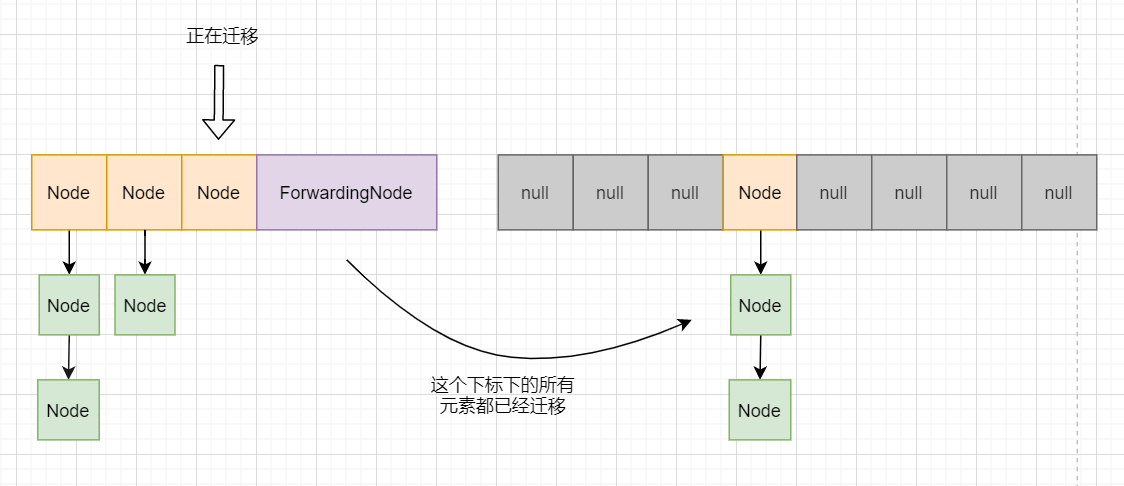ConcurrentHashMap会从后往前遍历并迁移，已经迁移完成的节点会被赋值为ForwardingNode，表示该节点下的所有数据已经迁移完成。ForwardingNode和普通的节点相似，但他的hash值为`MOVED`，也就是-1。还记得前面putVal吗？在插入的时候会判断当前节点是否是ForwardingNode，如果是则先帮忙迁移；否则如果正在扩容，说明扩容工作还没到达当前下标，那么可以直接插入。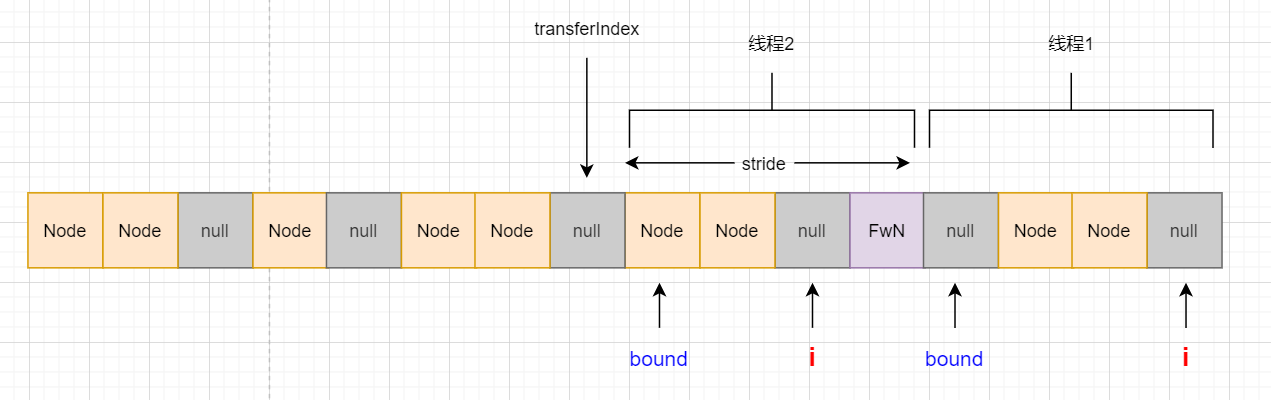ConcurrentHashMap把整个数组进行分段，每个线程负责一段。bound表示该线程范围的下限，i表示当前正在迁移的下标。每一个迁移完成的节点都会被赋值ForwardingNode，表示迁移完成。stride表示线程迁移的“步幅”，当线程完成范围内的任务后，就会继续往前看看还有没有需要迁移的，transferIndex就是记录下个需要迁移的下标；当transferIndex==0时则表示不需要帮忙了。这就是ConcurrentHashMap扩容方案的核心思路了 。保证线程安全的思路和前面介绍的方法大同小异，都是通过 CAS+自旋锁+synchronize来实现的。

``````private final void addCount(long x, int check) {
... // 总数+1逻辑

// 这部分的逻辑主要是判断是否需要扩容
// 同时保证只有一个线程能够创建新的数组
// 其他的线程只能辅助迁移数据
if (check >= 0) {
Node<K,V>[] tab, nt; int n, sc;
// 当长度达到阈值且长度并未达到最大值时进行下一步扩容
while (s >= (long)(sc = sizeCtl) && (tab = table) != null &&
(n = tab.length) < MAXIMUM_CAPACITY) {
// 这个数配合后续的sizeCtr计算
// 他的格式是第16位肯定为1,低15位表示n前面连续的0个数，我们前面介绍过
int rs = resizeStamp(n);
// 小于0表示正在扩容或者正在初始化,否则进入下一步抢占锁进行创建新数组
if (sc < 0) {
// 如果正在迁移右移16位后一定等于rs
// ( sc == rs + 1 ||sc == rs + MAX_RESIZERS)这两个条件我认为不可能为true
// 有兴趣可以点击下方网站查看
// https://bugs.java.com/bugdatabase/view_bug.do?bug_id=JDK-8214427
// nextTable==null说明下个数组还未创建
// transferIndex<=0说明迁移已经够完成了
// 符合以上情况的重新循环自旋
if ((sc >>> RESIZE_STAMP_SHIFT) != rs || sc == rs + 1 ||
sc == rs + MAX_RESIZERS || (nt = nextTable) == null ||
transferIndex <= 0)
break;
// 帮忙迁移,sc+1
if (U.compareAndSwapInt(this, SIZECTL, sc, sc + 1))
transfer(tab, nt);
}
// 抢占锁进行扩容
// 对rs检验码进行左移16位再+2，这部分我们在上面介绍过
else if (U.compareAndSwapInt(this, SIZECTL, sc,
(rs << RESIZE_STAMP_SHIFT) + 2))
// 抢占自旋锁成功，进行扩容
transfer(tab, null);

// 更新节点总数，继续循环
s = sumCount();
}
}
}``````

``````// 这里的两个参数：tab表示旧数组，nextTab表示新数组
// 创建新数组的线程nextTab==null，其他的线程nextTab等于第一个线程创建的数组
private final void transfer(Node<K,V>[] tab, Node<K,V>[] nextTab) {
int n = tab.length, stride;
// stride表示每次前进的步幅，最低是16
if ((stride = (NCPU > 1) ? (n >>> 3) / NCPU : n) < MIN_TRANSFER_STRIDE)
stride = MIN_TRANSFER_STRIDE; // subdivide range

// 如果新的数组还未创建，则创建新数组
// 只有一个线程能进行创建数组
if (nextTab == null) {
try {
@SuppressWarnings("unchecked")
// 扩展为原数组的两倍
Node<K,V>[] nt = (Node<K,V>[])new Node<?,?>[n << 1];
nextTab = nt;
} catch (Throwable ex) {
// 扩容失败出现OOM，直接把阈值改成最大值
sizeCtl = Integer.MAX_VALUE;
return;
}
// 更改concurrentHashMap的内部变量nextTable
nextTable = nextTab;
// 迁移的起始值为数组长度
transferIndex = n;
}

int nextn = nextTab.length;
// 标志节点，每个迁移完成的数组下标都会设置为这个节点
ForwardingNode<K,V> fwd = new ForwardingNode<K,V>(nextTab);
// finish表示迁移是否结束
// 官方的注释表示在赋值为true之前，必须再重新扫描一次确保迁移完成，后面会讲到
boolean finishing = false; // to ensure sweep before committing nextTab

// i表示当前线程迁移数据的下标，bound表示下限，从后往前迁移
for (int i = 0, bound = 0;;) {
Node<K,V> f; int fh;

// 这个循环主要是判断是否需要前进，如果需要则CAS更改下个bound和i
int nextIndex, nextBound;
if (--i >= bound || finishing)
// 每一轮循环更新transferIndex的下标
// 如果下一个下标是0，表示已经无需继续前进
else if ((nextIndex = transferIndex) <= 0) {
i = -1;
}
// 利用CAS更改bound和i继续前进迁移数据
else if (U.compareAndSwapInt
(this, TRANSFERINDEX, nextIndex,
nextBound = (nextIndex > stride ?
nextIndex - stride : 0))) {
bound = nextBound;
i = nextIndex - 1;
}
}

// i已经达到边界，说明当前线程的任务已经完成，无需继续前进
// 如果是第一个线程需要更新table引用
// 协助的线程需要将sizeCtl减一再退出
if (i < 0 || i >= n || i + n >= nextn) {
int sc;
// 如果已经更新完成，则更新table引用
if (finishing) {
nextTable = null;
table = nextTab;
// 同时更新sizeCtl为阈值
sizeCtl = (n << 1) - (n >>> 1);
return;
}
// 线程完成自己的迁移任务，将sizeCtl减一
if (U.compareAndSwapInt(this, SIZECTL, sc = sizeCtl, sc - 1)) {
// 这里sc-2不等于校验码，说明此线程不是最后一个线程，还有其他线程正在扩容
// 那么就直接返回，他任务已经完成了
// 最后一个线程需要重新把整个数组再扫描一次，看看有没有遗留的
if ((sc - 2) != resizeStamp(n) << RESIZE_STAMP_SHIFT)
return;
// finish设置为true表示已经完成
// 这里把i设置为n，重新把整个数组扫描一次
i = n; // recheck before commit
}
}
// 如果当前节点为null，表示迁移完成，设置为标志节点
else if ((f = tabAt(tab, i)) == null)
advance = casTabAt(tab, i, null, fwd);
// 当前节点是ForwardingNode，表示迁移完成，继续前进
else if ((fh = f.hash) == MOVED)
else {
// 给头节点加锁，进行迁移
// 加锁后下面的内容就不涉及并发控制细节了，就是纯粹的数据迁移
// 思路和HashMap差不多，但也有一些不同，多了一个lastRun
// 读者可以阅读一下下面源码，这部分比较容易理解
synchronized (f) {
// 上锁之后再判断一次看该节点是否还是原来那个节点
// 如果不是则重新循环
if (tabAt(tab, i) == f) {
Node<K,V> ln, hn;
// hash值大于等于0表示该节点是普通链表节点
if (fh >= 0) {
int runBit = fh & n;
Node<K,V> lastRun = f;
// ConcurrentHashMap并不是直接把整个链表分为两个
// 而是先把尾部迁移到相同位置的一段先拿出来
// 例如该节点迁移后的位置可能为 1或5 ，而链表的情况是：
// 1 -> 5 -> 1 -> 5 -> 5 -> 5
// 那么concurrentHashMap会先把最后的三个5拿出来，lastRun指针指向倒数第三个5
for (Node<K,V> p = f.next; p != null; p = p.next) {
int b = p.hash & n;
if (b != runBit) {
runBit = b;
lastRun = p;
}
}
// 判断尾部整体迁移到哪个位置
if (runBit == 0) {
ln = lastRun;
hn = null;
}
else {
hn = lastRun;
ln = null;
}
for (Node<K,V> p = f; p != lastRun; p = p.next) {
int ph = p.hash; K pk = p.key; V pv = p.val;
// 这个node节点是改造过的
// 相当于使用头插法插入到链表中
// 这里的头插法不须担心链表环，因为已经加锁了
if ((ph & n) == 0)
ln = new Node<K,V>(ph, pk, pv, ln);
else
hn = new Node<K,V>(ph, pk, pv, hn);
}
// 链表构造完成，把链表赋值给数组
setTabAt(nextTab, i, ln);
setTabAt(nextTab, i + n, hn);
// 设置标志对象，表示迁移完成
setTabAt(tab, i, fwd);
}
// 树节点的处理，和链表思路相同，不过他没有lastRun，直接分为两个链表，采用尾插法
else if (f instanceof TreeBin) {
TreeBin<K,V> t = (TreeBin<K,V>)f;
TreeNode<K,V> lo = null, loTail = null;
TreeNode<K,V> hi = null, hiTail = null;
int lc = 0, hc = 0;
for (Node<K,V> e = t.first; e != null; e = e.next) {
int h = e.hash;
TreeNode<K,V> p = new TreeNode<K,V>
(h, e.key, e.val, null, null);
if ((h & n) == 0) {
if ((p.prev = loTail) == null)
lo = p;
else
loTail.next = p;
loTail = p;
++lc;
}
else {
if ((p.prev = hiTail) == null)
hi = p;
else
hiTail.next = p;
hiTail = p;
++hc;
}
}
ln = (lc <= UNTREEIFY_THRESHOLD) ? untreeify(lo) :
(hc != 0) ? new TreeBin<K,V>(lo) : t;
hn = (hc <= UNTREEIFY_THRESHOLD) ? untreeify(hi) :
(lc != 0) ? new TreeBin<K,V>(hi) : t;
setTabAt(nextTab, i, ln);
setTabAt(nextTab, i + n, hn);
setTabAt(tab, i, fwd);
}
}
}
}
}
}``````

``````final Node<K,V>[] helpTransfer(Node<K,V>[] tab, Node<K,V> f) {
Node<K,V>[] nextTab; int sc;
// 判断当前节点为ForwardingNode，且已经创建新的数组
if (tab != null && (f instanceof ForwardingNode) &&
(nextTab = ((ForwardingNode<K,V>)f).nextTable) != null) {
int rs = resizeStamp(tab.length);
// sizeCtl<0表示还在扩容
while (nextTab == nextTable && table == tab &&
(sc = sizeCtl) < 0) {
// 校验是否已经扩容完成或者已经推进到0，则不需要帮忙扩容
if ((sc >>> RESIZE_STAMP_SHIFT) != rs || sc == rs + 1 ||
sc == rs + MAX_RESIZERS || transferIndex <= 0)
break;
// 尝试让让sc+1并帮忙扩容
if (U.compareAndSwapInt(this, SIZECTL, sc, sc + 1)) {
transfer(tab, nextTab);
break;
}
}
// 返回扩容之后的数组
return nextTab;
}
// 若数组尚未初始化或节点非ForwardingNode,返回原数组
return table;
}``````